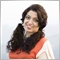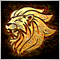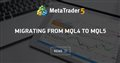# how to change iMAOnArray from Mt4 to MT5182

hi every one

I used below code in my expert in MT4 now I want use it in MT5 what should I replace instead of iMAOnArray in MT5

` SignalBuffer[i]=iMAOnArray(MainBuffer,Bars,DPeriod,0,MODE_SMA,i);`

best regrads

NedaModerator
13655Migrating from MQL4 to MQL5
• www.mql5.com
Practical Application Of Databases For Markets Analysis Working with data has become the main task for modern software - both for standalone and network applications. To solve this problem a specialized software were created. These are Database Management Systems (DBMS), that can structure, systematize and organize data for their computer...36

Neda shahbazi:

hi every one

I used below code in my expert in MT4 now I want use it in MT5 what should I replace instead of iMAOnArray in MT5

best regrads

Neda

```double iMAOnArrayMQL4(double &array[],
int total,
int period,
int ma_shift,
int ma_method,
int shift)
{
double buf[],arr[];
if(total==0) total=ArraySize(array);
if(total>0 && total<=period) return(0);
if(shift>total-period-ma_shift) return(0);
switch(ma_method)
{
case MODE_SMA :
{
total=ArrayCopy(arr,array,0,shift+ma_shift,period);
if(ArrayResize(buf,total)<0) return(0);
double sum=0;
int    i,pos=total-1;
for(i=1;i<period;i++,pos--)
sum+=arr[pos];
while(pos>=0)
{
sum+=arr[pos];
buf[pos]=sum/period;
sum-=arr[pos+period-1];
pos--;
}
return(buf);
}
case MODE_EMA :
{
if(ArrayResize(buf,total)<0) return(0);
double pr=2.0/(period+1);
int    pos=total-2;
while(pos>=0)
{
if(pos==total-2) buf[pos+1]=array[pos+1];
buf[pos]=array[pos]*pr+buf[pos+1]*(1-pr);
pos--;
}
return(buf[shift+ma_shift]);
}
case MODE_SMMA :
{
if(ArrayResize(buf,total)<0) return(0);
double sum=0;
int    i,k,pos;
pos=total-period;
while(pos>=0)
{
if(pos==total-period)
{
for(i=0,k=pos;i<period;i++,k++)
{
sum+=array[k];
buf[k]=0;
}
}
else sum=buf[pos+1]*(period-1)+array[pos];
buf[pos]=sum/period;
pos--;
}
return(buf[shift+ma_shift]);
}
case MODE_LWMA :
{
if(ArrayResize(buf,total)<0) return(0);
double sum=0.0,lsum=0.0;
double price;
int    i,weight=0,pos=total-1;
for(i=1;i<=period;i++,pos--)
{
price=array[pos];
sum+=price*i;
lsum+=price;
weight+=i;
}
pos++;
i=pos+period;
while(pos>=0)
{
buf[pos]=sum/weight;
if(pos==0) break;
pos--;
i--;
price=array[pos];
sum=sum-lsum+price*period;
lsum-=array[i];
lsum+=price;
}
return(buf[shift+ma_shift]);
}
default: return(0);
}
return(0);
}```1660

See also the MovingAverages.mqh in your MT5 include folder. It contains several functions to calculate a moving average from a buffer:

```int SimpleMAOnBuffer(const int rates_total,const int prev_calculated,const int begin,
const int period,const double& price[],double& buffer[])```
```int ExponentialMAOnBuffer(const int rates_total,const int prev_calculated,const int begin,
const int period,const double& price[],double& buffer[])```
```int LinearWeightedMAOnBuffer(const int rates_total,const int prev_calculated,const int begin,
const int period,const double& price[],double& buffer[],int &weightsum)```
```int SmoothedMAOnBuffer(const int rates_total,const int prev_calculated,const int begin,
const int period,const double& price[],double& buffer[])```